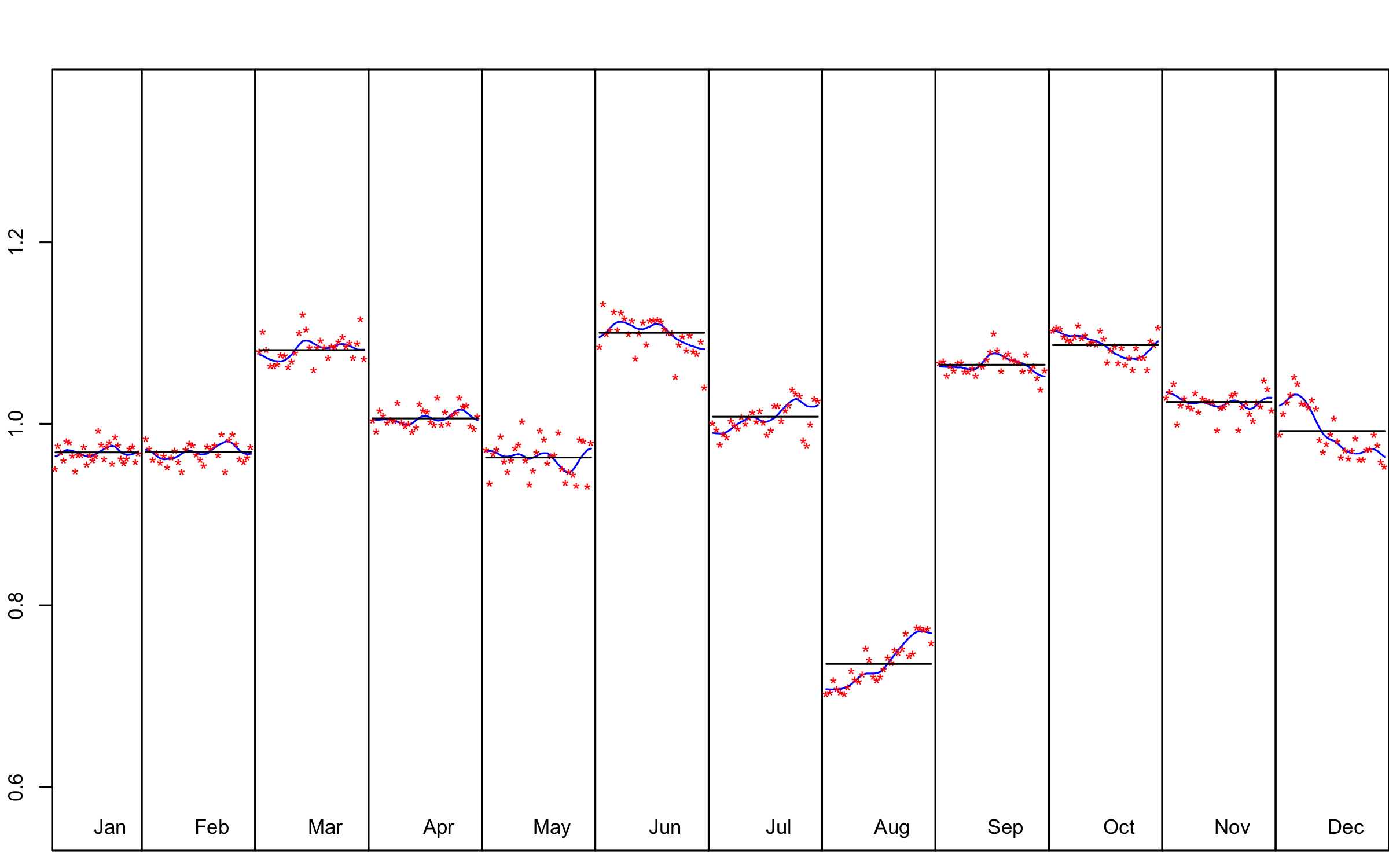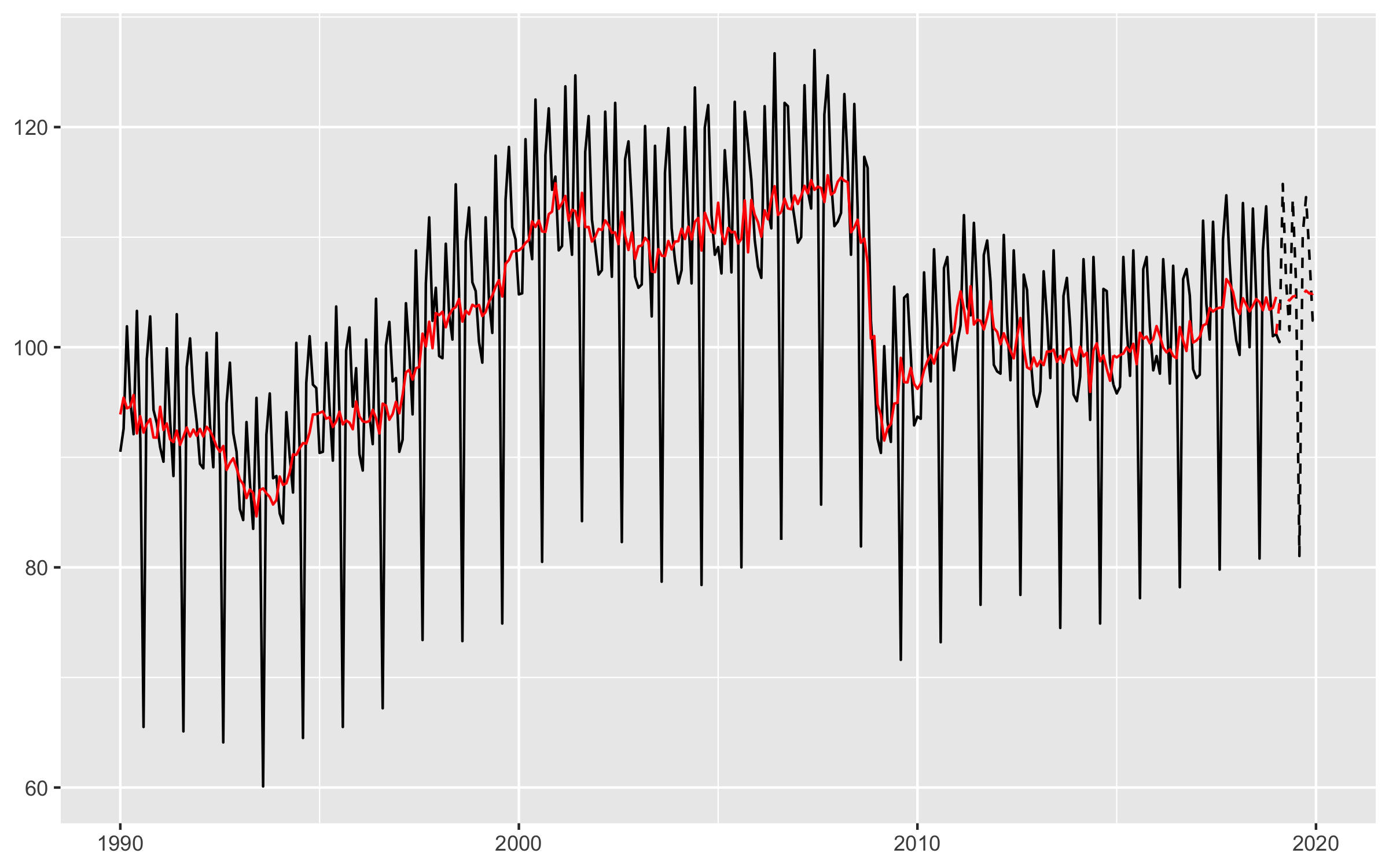# rjdmarkdown with HTML output

The functions developped in `rjdmarkdown` are:

• `print_preprocessing()` for the pre-processing model;
• `print_decomposition()` for the decomposition;
• `print_diagnostics()` to print diagnostics tests on the quality of the seasonal adjustment.

The result is different between X-13ARIMA and TRAMO-SEATS models.

``````library(rjdmarkdown)
library(RJDemetra)
sa_x13 <- x13(ipi_c_eu[, "FR"])
sa_ts <- tramoseats(ipi_c_eu[, "FR"])``````

# X-13-ARIMA model

``print_preprocessing(sa_x13)``

Pre-processing (RegArima)

Summary

360 observations

Series has been log-transformed

Trading days effect (6 variables)

Easter  detected

3 detected outliers

Likelihood statistics

Number of effective observations = 347

Number of estimated parameters = 15

Loglikelihood = 853.667, AICc = 1529.651, BICc = -7.547

Standard error of the regression (ML estimate) = 0.020

ARIMA model

ARIMA coefficients
Coefficients Std. Error T-stat P (> | t|)
Phi(1) 0.026 0.118 0.218 0.827
Phi(2) 0.159 0.078 2.039 0.042
Theta(1) -0.517 0.113 -4.589 0.000 ***
BTheta(1) -0.703 0.041 -17.093 0.000 ***
Signif. codes: 0 ‘***’ 0.001 ‘**’ 0.01 ‘*’ 0.05 ‘.’ 0.1 ’ ’ 1
ARIMA (2,1,1)(0,1,1)

Regression model

Regression coefficientss
Coefficients Std. Error T-stat P (> | t|)
Monday 0.005 0.002 2.309 0.022
Tuesday 0.008 0.002 3.895 0.000 ***
Wednesday 0.011 0.002 4.903 0.000 ***
Thursday 0.002 0.002 0.955 0.340
Friday 0.008 0.002 3.826 0.000 ***
Saturday -0.016 0.002 -7.599 0.000 ***
Easter  -0.021 0.004 -4.979 0.000 ***
AO (5-2011) 0.128 0.017 7.447 0.000 ***
LS (11-2008) -0.087 0.017 -5.131 0.000 ***
LS (1-2009) -0.068 0.017 -4.037 0.000 ***
Signif. codes: 0 ‘***’ 0.001 ‘**’ 0.01 ‘*’ 0.05 ‘.’ 0.1 ’ ’ 1
``print_decomposition(sa_x13, caption = NULL)``

Decomposition (X-11)

Mode: multiplicativeS-I Ratio

M-statistics
Value Description
M-1 0.097 The relative contribution of the irregular over three months span
M-2 0.053 The relative contribution of the irregular component to the stationary portion of the variance
M-3 0.995 The amount of period to period change in the irregular component as compared to the amount of period to period change in the trend
M-4 0.421 The amount of autocorrelation in the irregular as described by the average duration of run
M-5 0.950 The number of periods it takes the change in the trend to surpass the amount of change in the irregular
M-6 0.154 The amount of year to year change in the irregular as compared to the amount of year to year change in the seasonal
M-7 0.074 The amount of moving seasonality present relative to the amount of stable seasonality
M-8 0.211 The size of the fluctuations in the seasonal component throughout the whole series
M-9 0.064 The average linear movement in the seasonal component throughout the whole series
M-10 0.267 The size of the fluctuations in the seasonal component in the recent years
M-11 0.250 The average linear movement in the seasonal component in the recent years
Q 0.322
Q-M2 0.355
Final filters: M3x5, Henderson-13 terms
Relative contribution of the components to the stationary portion of the variance in the original series, after the removal of the long term trend
Component
Cycle 1.941
Seasonal 62.123
Irregular 0.842
TD & Hol. 2.428
Others 32.787
Total 100.121
``print_diagnostics(sa_x13)``
Diagnostics tests
P (> | t|)
mean 0.797
skewness 0.383
kurtosis 0.409
ljung box 0.000 ***
ljung box (residuals at seasonal lags) 0.199
ljung box (squared residuals) 0.802
qs test on sa 0.909
qs test on i 0.746
f-test on sa (seasonal dummies) 0.974
f-test on i (seasonal dummies) 0.947
Residual seasonality (entire series) 0.932
Residual seasonality (last 3 years) 0.940
f-test on sa (td) 0.985
f-test on i (td) 0.983
Signif. codes: 0 ‘***’ 0.001 ‘**’ 0.01 ‘*’ 0.05 ‘.’ 0.1 ’ ’ 1

# TRAMO-SEATS model

Some others graphics can also be added with the `ggdemetra` package, for example to add the seasonally adjusted series and its forecasts:

``````library(ggdemetra)
ggplot(data = ipi_c_eu_df, mapping = aes(x = date, y = FR)) +
geom_line() +
labs(title = NULL,
x = NULL, y = NULL) +
geom_sa(component = "y_f", linetype = 2,
frequency = 12, method = "tramoseats") +
geom_sa(component = "sa", color = "red") +
geom_sa(component = "sa_f", color = "red", linetype = 2)``````Seasonal adjustment of the French industrial production index

``print_preprocessing(sa_ts)``

Pre-processing (Tramo)

Summary

360 observations

Series has been log-transformed

Trading days effect (2 variables)

Easter  detected

2 detected outliers

Likelihood statistics

Number of effective observations = 347

Number of estimated parameters = 9

Loglikelihood = 831.828, AICc = 1560.412, BICc = -7.523

Standard error of the regression (ML estimate) = 0.022

ARIMA model

ARIMA coefficients
Coefficients Std. Error T-stat P (> | t|)
Phi(1) 0.386 0.053 7.287 0.000 ***
Phi(2) 0.243 0.053 4.579 0.000 ***
BTheta(1) -0.713 0.040 -17.672 0.000 ***
Signif. codes: 0 ‘***’ 0.001 ‘**’ 0.01 ‘*’ 0.05 ‘.’ 0.1 ’ ’ 1
ARIMA (2,1,0)(0,1,1)

Regression model

Regression coefficientss
Coefficients Std. Error T-stat P (> | t|)
Week days 0.007 0.000 23.891 0.000 ***
Leap year 0.022 0.007 3.238 0.001 **
Easter  -0.021 0.004 -5.118 0.000 ***
AO (5-2011) 0.129 0.017 7.640 0.000 ***
AO (5-2000) 0.058 0.017 3.409 0.001 ***
Signif. codes: 0 ‘***’ 0.001 ‘**’ 0.01 ‘*’ 0.05 ‘.’ 0.1 ’ ’ 1
``print_decomposition(sa_ts, caption = NULL)``

Decomposition (SEATS)

Mode: multiplicative